# Higgs Decays to Unknown Spin-Zero Particles

On this page, I’ll be describing (following our recent paper; experts, see also our technical website) what can happen if the newly discovered Standard Model-like Higgs particle can decay to a pair of particles which have spin-zero, and then these in turn each decay to two known particles.  There are a few common patterns which I’ll discuss, and I’ll point out the searches that we think are the most promising, both now, using existing Large Hadron Collider [LHC] data, and in the near future, when the LHC starts running again in 2015.

Nontechnical Aside

A few notes for non-experts:

Symbols: “b” = bottom quark, “τ” – tau lepton, “μ” = muon,  “γ” = photon, “g” = gluon.

The new spin-zero particle a to which the Higgs is assumed to decay is a “singlet”: a field and/or particle that is not affected by the known non-gravitational forces: the electromagnetic, strong nuclear, and weak nuclear forces.

Branching fraction: If you make a Higgs particle, the probability that it decays to two photons is called “the branching fraction for h → γγ”, or “Br(h→ γγ)”.

Technical Introduction

The decay of the 125 GeV Higgs particle to two spin-zero particles (scalars or pseudo-scalars, generically called “a” below) is predicted to occur in a wide variety of speculative theories with extra Higgs fields.  These theories include those with two Higgs doublets and one or more Higgs singlets, such as (but not limited to) the Next-to-Minimal Supersymmetric Standard Model (NMSSM).  It also often occurs in models with just one Higgs doublet and singlets.  The singlets in question may be elementary, or they may be composite (as in Little Higgs models or in Hidden Valleys.)

Because the Higgs field H has dimension 1 and its square has dimension 2, fundamental scalar fields S can interact with it at the renormalizable level, for instance (in the case of a real scalar) via the interaction ξ|H|² S².  Once the H field’s average value is non-zero, then a decay of the Higgs particle h to S particles, h → SS, may occur, if the S mass is less than 62 GeV/c².  In models with a complex scalar, decays to pseudo-scalars a may also easily occur.  (Composite [pseudo-]scalars are a more elaborate subject, but the observable decays are the same.)  Even for small ξ, this decay can in principle reach nearly 100%.  Of course, that is now ruled out experimentally by the observation of a Standard Model-like Higgs, but the decay in question could still occur with branching fraction anywhere below the current experimental bound, which is between about 20% and 50%, depending on what assumptions are made.

If the symmetry S ↔ -S is exact or nearly so, then S will be stable (or nearly so) and so the decay will be unobservable (i.e. “invisible”).  Searches for invisible decays have reached 68% branching fraction, and may reach sensitivity approaching 20% in the coming run. However, if the symmetry S ↔ -S is explicitly or spontaneously broken, then h and S will mix and decays of the S that mimic those of a very light Higgs particle, dominated by the heaviest available flavors of quarks and leptons. Similar phenomenology arises in many models with pseudo-scalars. If carried out, searches for such decays could in some cases reach below 10% even with current 2011-2012 data.  We’ll see this in a moment.

Decays to Quarks and Leptons

In a model like the NMSSM, the decay rates of the a, like those of the Higgs h itself, are generally proportional to the masses m of the particles to which it decays.  In this case, if ma > 2 mb, the pattern of decays is approximately as shown in Figure 1.  [Be sure to interpret this properly! If h → aa has a branching fraction of 0.03, then the branching fraction of h → aa → (bb)(μμ) is 0.03 × (3×10-4) = 1×10-5.]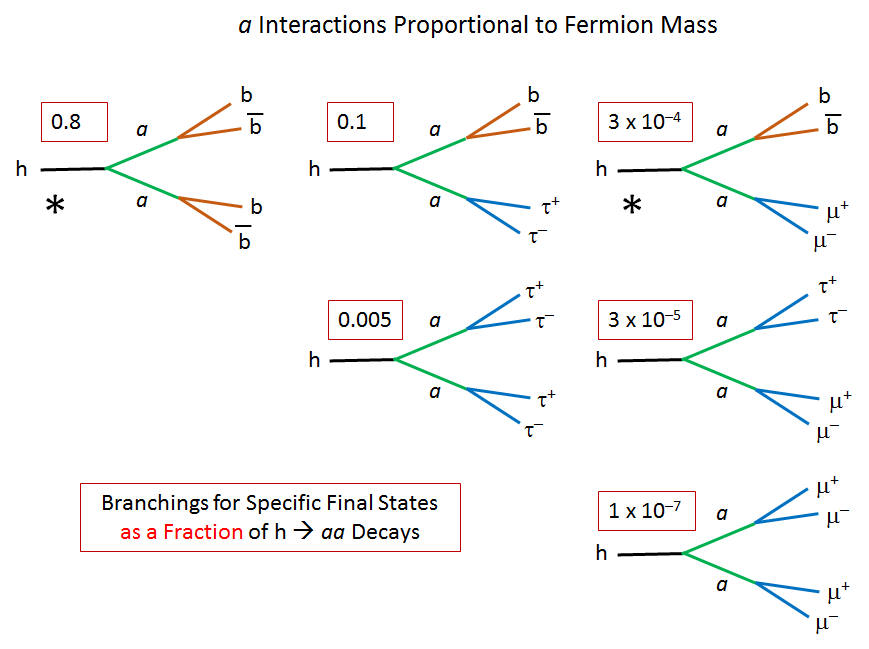Fig. 1: Pattern of decays if h → aa and a → quarks and leptons proportional to their masses. Multiply the numbers shown by the branching fraction for h → aa to get the Higgs branching fractions. The most promising decays for searches in post-2015 data are indicated by an asterisk.

We have studied all of these decays to greater or lesser degrees, and it appears the two best channels are h→(bb)(bb) (where the Higgs is produced in pp → W+h or Z+h ) and h→ (bb)(μμ) (where the Higgs is produced in any mode).  Both come into their own in 2015 and beyond; see Figure 2, which shows an optimistic scenario for h→ (bb)(μμ) in current data.  We should note, however, that h→ (bb)(ττ) might be as powerful as h→ (bb)(bb) if quark couplings of the a are somewhat suppressed relative to its lepton couplings.  This decay deserves more study by both theorists and experimentalists.Fig. 2: Estimates for a search, using current data, for h → (bb)(μμ), based on calculation. Top: estimate of the di-muon mass spectrum for backgrounds, and a possible signal (in red) if the branching fraction for h → aa is 10%; left with at least one b-tagged jet, right with two b-tagged jets. Bottom: estimate of possible limit on h → aa → (bb)(μμ).

What Figure 2 shows is that the decay h → aa → (bb)(μμ) produces a narrow peak in the invariant mass distribution of the muon/anti-muon pair, at the mass of the a.  [Similar peaks allowed for the discovery of the Higgs itself.]  But notice the number of events expected is small.  It appears current data isn’t quite enough to reach h → aa at the 10% level, though the data to come in 2015-2018 will be more than enough.

Decays Without Bottom Quarks, Mainly To Leptons

However, if

• ma < 2 mb, so decays to bottom quarks are impossible, or
• a interacts much more strongly with leptons than with quarks, as occurs in certain two-Higgs doublet models with a singlet Higgs (specifically “Type III”, at moderate to large tan β; see section 1.3.2 of  our paper, arxiv.org/abs/1312.4992)

then the usually-dominant decays to quarks may be suppressed or absent.  If decays are only to leptons, then the decay pattern is as shown in Figure 3.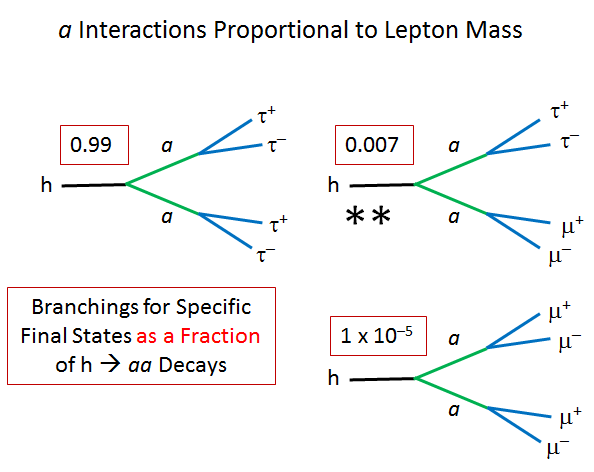Fig. 3: As in Figure 1, but with decays to quarks suppressed, either because a→ bb is not allowed kinematically or because a interacts weakly with quarks. The decay h → aa → (ττ)(μμ) is marked with a double-asterisk because a search for it in 2011-2012 data would put very interesting limits on h → aa. See Figure 4.

Our reinterpretation of existing multi-lepton data as a search for h → aa → (ττ)(ττ) and (ττ)(μμ) only allows us [Figure 4, top] to infer limits on h → aa of order 20%.  But the decay h → aa → (ττ)(μμ) has a muon/anti-muon resonance peak [like the bump seen in Figure 2].  If one searches directly for this peak, we estimate this may provide (Figure 4, bottom) a limit on h → aa of 2 – 10% with current data. Thus a dedicated search for h → (ττ)(μμ) is highly motivated!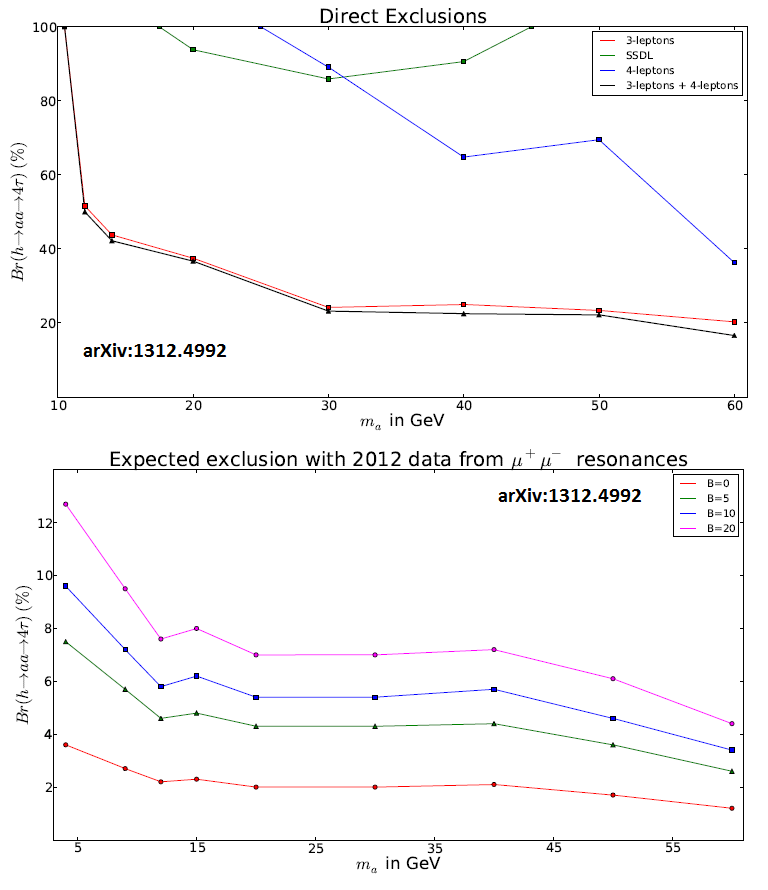Fig. 4: Limits, using current 2011-2012 data, on h→ aa (assuming a dominantly decays to ττ, with a small subleading decay to μμ as in Figure 3). Top: our reinterpretation of existing multi-lepton searches (red shows CMS 3-leptons, blue CMS 4-leptons, green ATLAS same-sign dileptons, grey the combination of the first two channels) already put limits on h→ aa in the 20-40% range. Bottom: our estimate of the possible capability of a search for the muon/anti-muon mass peak (see Figure 2) in h→ aa→ (ττ)(μμ). Since we cannot estimate the backgrounds, we present limits for various possibilities: the four curves assume a different number B of background events under the resonance peak, ranging across 0, 5, 10 and 20. The lowest curve (B=0) represents the best possible result, roughly 2% – 3% limits on h → aa.

Decays to Gluons and Photons

In other speculative theories that go beyond the Standard Model, there can be new low-mass spin-zero singlet particles a that interact very weakly with the known fermions (i.e. the so-called “matter particles”: quarks, leptons and neutrinos).  [Here’s an example of such a theory.] In this case, it may be that the interaction of a with Standard Model particles occurs through as-yet unknown fields with high-mass particles, called “R” in Figure 5, that feel the electromagnetic, strong or weak nuclear forces, and also interact with the a fields. Consequently, R `virtual particles‘ (i.e. generalized disturbances in the R fields) will induce new interactions of a type that may allow the new a particles to decay to a pair of gluons or photons. (This is similar to how the Higgs interacts indirectly with photons and gluons, as a result of virtual top quarks and W particles.)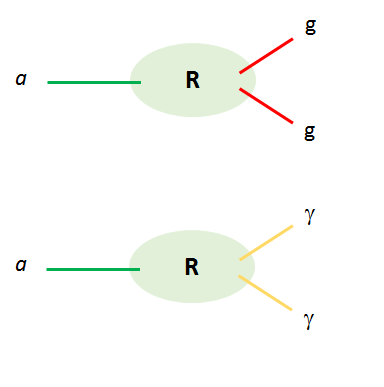Fig. 5: If there are unknown fields R whose particles are very heavy, their “virtual particles” can induce an indirect interaction between the a particles and pairs of photons and/or gluons. Also, a coupling to one Z and one photon is present, but generally too small to be important, while couplings to W and Z pairs are unimportant due to the a‘s small mass.

[Note: We won’t see decays to W and Z particles.  If an “a” particle is sufficiently light that it can appear in the decay of the Higgs, then it is too light to decay to a pair of W or Z particles, and virtual W/Z particles will not appear with a measurable rate.]

If the particles R are affected by both electromagnetic and strong nuclear forces, and all the R particles have the same mass, then the decay of the a to photons and gluons is proportional to the strength of the corresponding force (times 8 for the gluons, since there are eight types of gluons). In that case, the pattern of exotic decays of the Higgs particle will be typically as shown in Figure 6. [Experts; this is for a complete SU(5) quintuplet.] In this case, the decay h → aa → four-photons is perhaps the most promising for a clear discovery in the very long run, because backgrounds are extremely small; but with so few events this would likely not be observable for the foreseeable future.  (These studies should be revisited, however.)  Instead, studies in the literature suggest that is also possible that the four-gluon final state (which leads to at most four distinguishable jets [sprays of hadrons]) will be observable in the distant future, in events where the Higgs is produced along with a W or Z particle.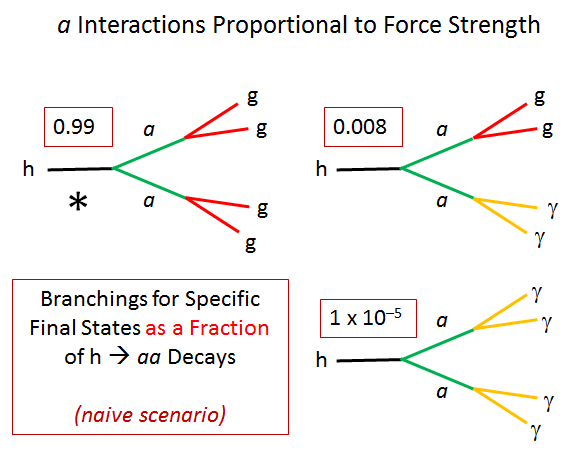Fig. 6: If the interactions in Figure 5 are present but the interactions of a with quarks, leptons and neutrinos are small, then the new decays of the h will be as shown. The numbers show the relative branching fractions for a simple case described in the text. (Remember to multiply by the branching fraction for h → aa to get the full h → 4 photon branching fraction.) Decays to four gluons will someday be promising, but not soon, and only if the branching fraction for h → aa is in the 10% range; hence a single asterisk. Decays to four photons are very rare; however, see Figure 7.

However, it is not unusual or unexpected for some of the particles R to be ones that are not affected by the strong nuclear force.  It is also common for those particles to be significantly lighter than those that are affected by the strong nuclear force. If this is the case, then the decay of a to photons is enhanced, and the probability of a four-photon decay can increase sharply; see Figure 7. In this case, theoretical studies suggest that a search for the four-photon decay of the Higgs particle in existing data could put a limit on the branching fraction for h → aa at 20%.   Again the four-gluon decay would eventually be detectable many years from now.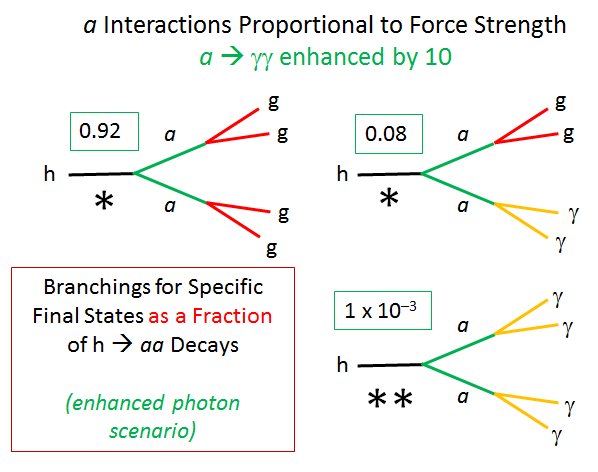Fig. 7: As in Figure 6, but when the R particles with strong nuclear forces are heavier than those without them, leading to an enhancement of a → γγ by a factor of 10. Then the decay to four photons is potentially observable now or in the relatively near future (hence, two asterisks).As in Figure 6, the four-jet final state may eventually reach observable levels, and the decay to two photons and two jets may someday be observable, if h → aa is 10%-20% (one asterisk).

[Studies in the literature suggest that the two-photon/two-gluon decay (giving two photons and one or two jets in the detector) would potentially be observable in the far future, but we think these probably would not be competitive with the others — unless the two pseudoscalars in the Higgs decay are actually different particles with different masses and branching fractions.]

Of course, it could also be that the R particles that interact with a are affected only by electromagnetic forces, and not by strong nuclear forces.  In that case there would be no decays to gluons, and all decays would be to photons, potentially making h → aa → (γγ)(γγ) easily discoverable with existing data, with limits reaching a branching fraction for h → aa of 0.002.

As far as we know, there are currently no limits on these processes that can currently be inferred from published LHC data.

In summary, a search for h → aa → (γγ)(γγ) is already motivated with current data.  In the long run, a search for h → aa → (gg)(gg), in events where a W or Z is produced along with the Higgs, will also be needed.

### 4 thoughts on “Higgs Decays to Unknown Spin-Zero Particles”

This site uses Akismet to reduce spam. Learn how your comment data is processed.